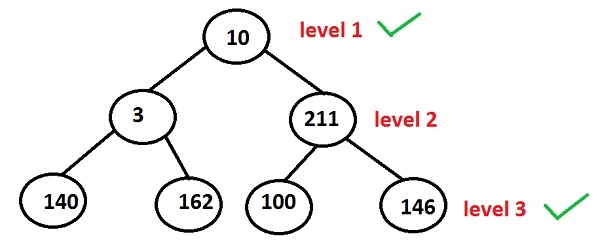# Print the nodes at odd levels of a tree in C++ Programming.

Given the binary tree, the program must print the nodes at odd levels of a tree and the levels of a binary tree start from 1 to n.

As nothing is mentioned one of the two approaches can be implemented i.e. recursion or iteration.

Since we are using a recursive approach, the program will make a recursive call to a function that will be fetching the nodes at odd levels and returning them.In the above binary tree −

Nodes at level 1: 10
Nodes at level 2: 3 and 211
Nodes at level 3: 140, 162, 100 and 146

So, the nodes at level 1 and level 3 will be printed that means the output will be 10, 140, 162, 100 and 146.

## Algorithm

START
Step 1 -> create a structure of a node as
struct Node
struct node *left, *right
int data
End
Step 2 -> function to create a node
node* newnode(int data)
node->data = data
node->left = node->right = NULL;
return (node)
step 3 -> create function for finding the odd nodes
void odd(Node *root, bool ifodd = true)
IF root = NULL
Return
End
if (ifodd)
print root->data
End
odd(root->left, !ifodd)
odd(root->right, !ifodd)
step 4 -> In main()
Create tree using Node* root = newnode(45)
root->left = newnode(23)
Call odd(root)
Stop

## Example

Live Demo

#include <bits/stdc++.h>
using namespace std;
struct Node{
int data;
Node* left, *right;
};
void odd(Node *root, bool ifodd = true){
if (root == NULL)
return;
if (ifodd)
cout << root->data << " " ;
odd(root->left, !ifodd);
odd(root->right, !ifodd);
}
// function to create a new node
Node* newnode(int data){
Node* node = new Node;
node->data = data;
node->left = node->right = NULL;
return (node);
}
int main(){
Node* root = newnode(45);
root->left = newnode(23);
root->right = newnode(13);
root->left->left = newnode(24);
root->left->right = newnode(85);
cout<<"\nodd nodes are ";
odd(root);
return 0;
}

## Output

if we run the above program then it will generate the following output

odd nodes are 45 24 85